# A generalized correlation for the estimation of moisture removal in fruits and grains during hot air drying

A generalized correlation for the estimation of moisture removal in fruits and grains during hot air drying

Rama Krishna Konijeti Pullela Kameswara Sarma*Naveen Puppala K.V. Sharma L.S.V. Prasad

GITAM University, Beach Road, Gandhi Nagar, Rushikonda, Visakhapatnam, Andhra Pradesh, 53004, India

College of Agricultural, Consumer and Environmental Sciences, Agricultural Science Center Clovis 2346, State Road 288 Clovis, New Mexico, 88101-9998, USA

Centre for Energy Studies, JNTUH, Kukatpally, Hyderabad, Telangana, 500085, India

Associate Professor, Andhra University, Waltair Junction, Visakhapatnam, Andhra Pradesh 530003, India

Corresponding Author Email:
sarmapk@yahoo.com
Page:
426-432
|
DOI:
https://doi.org/10.18280/ijht.350228
|
Accepted:
|
Published:
30 June 2017
| Citation

OPEN ACCESS

Abstract:

Drying of food and grain material for removal of moisture is an important aspect in preserving them or for extraction of oil. It is a complex process involving both heat and mass transfer. The present work is aimed at developing a simple mathematical model with governing differential equations and proper boundary conditions for spherical shaped food material to determine the rate of moisture removal. The differential equations are solved using numerical technique with the help of a computer Program and the results are presented in graphical form. The variation of temperature within the seed with respect to time for various drying medium temperatures considering the partial pressure of water vapor in the drying medium are presented. The rate of moisture removal with respect to time for different convective environment conditions and varying resistance within the seed is also presented. A generalized correlation to determine the moisture rate removal as a function of Fourier number, Biot number and Mass transfer Parameter is developed for estimation of time required for heating under different hot air conditions for various spherical shaped fruits or grains. This non-dimensional correlation is helpful in quick estimation of time for drying of any seed and hence would be useful in the Agro Industry.

Keywords:

mass transfer, unsteady state, biot number, fourier number, moisture

1. Introduction

Artificial drying of food and seeds has gain importance in Agro and food industry to preserve them for a long time, and is considered as an energy intensive process. There is an ever-demanding growth in the proper utilization of surplus crop to meet the requirements during un-season. Unless proper drying procedures are adopted storage and quality perseverance is impossible. Understanding and controlling the drying mechanism is important in the design of heated air dryers with optimum heating temperature and time otherwise it may lead to the damage of products. During the process, the seed is heated by using hot medium giving rise to temperature gradient and moisture gradient within the seed. The moisture gradient between the seed and surrounding medium causes migration of moisture from seed. The humidity and temperature of heating medium is also important in attaining the equilibrium moisture content of the seed as desirable. As it involves both heat and Mass transfer the kinetics involved in this process are so complicate. As all the food grains do not possess same amount of moisture and the atmospheric conditions in which they are subjected to drying being not same and the estimation of time requirement varies from one to the other. Luikov  has formulated one dimensional system of equations to simulate the temperature and moisture profile during the drying seeds. However, its application was limited to only few varieties of seeds.

A two-dimensional finite element model was developed to simulate moisture diffusion into a corn kernel by Muthukumarappan and Gunasekaran . They observed that the rate of absorption in first one hour is high and subsequently declined. Ranjan et al.  have presented a set of one way coupled heat and diffusive moisture transfer equations by assuming that the moisture diffuses to the outer boundaries of the food material in liquid form and that evaporation takes place only at the surface of the food material. However, the model was solved in three–dimensional Cartesian coordinate system which is uncommon for fruits and seeds. To account for the irregular shape of seeds Agrawal et al.  have developed different models to analyze various geometries to estimate projected area, surface area and volume. They considered Ellipsoid and Cassinoid geometry models which divide a random sample approximately in two halves.

Haghighi et al.  developed set of coupled conductive heat and diffusive moisture transfer equations to simulate drying of axisymmetric grains. The model considers the temperature and moisture dependence of the diffusion coefficient, thermal conductivity, and specific heat. The results obtained by finite element analysis are compared with the experimental data of barley kernel and claimed to be useful for grain quality evaluation and drying simulation studies. However, the solution is limited only to barley kernel. Manal and Mohammed Studied  air drying of a single kernel fruit like apple, apricot and grape considering convective heat and mass transfer between the sample surface and its drying environment under unsteady heat conduction. They assumed that the moisture diffusion take place within the drying fruit without any phase change. The governing equations were solved by using the finite difference technique. A set of empirical correlations have been employed to determine the thermal conductivity, specific heat and coefficient of moisture diffusion. They found that in the drying process the temperature of the fruit is increased and its moisture content is decreased. The numerical results were compared satisfactorily with experimental results. Shrinkage and porosity effects are studied during potato drying by Aprajeeta et al.  Subjecting Potato slices to drying. They observed that shrinkage varies linearly with respect to moisture content and reduction in radial dimension of potato slices further the porosity undergoes rapid increase after attaining certain moisture content in the final stages of drying.

Stanescu et al.  have addressed the fundamental problem of determining best strategies to minimize the specific energy and the mass flow rate of drying gas for moisture removal from grains. Mihoubi and Bellagi  developed a relatively simple two-dimensional drying model which takes into account deformation of the dried material to analyze the spatio-temporal distribution of the temperature, the moisture and the solid displacement during the process. The model assumes a homogeneous, hygroscopic, isotropic and highly shrinkable medium and was solved numerically by a finite difference method.

Aviara et al.  have compared the five commonly used moisture sorption isotherm models to predict the equilibrium moisture content and its temperature dependence of soya bean. They identified that the heat of vaporization of moisture in soya bean decreased with increase in moisture content and the surface potential (spreading pressure) of moisture in the seed increased with increase in water activity and the effect of temperature was not much significant. Muchilwa et al.  considered thin layer drying film models for accurate drying time prediction considering variable diffusivity and recommended field-based rapid profiling of product drying, as opposed to universal equation modeling. The drying kinetics of the red bell pepper was studied and modeled at different temperatures at a specified air velocity by Vega et al.  The effect of temperature on the diffusivity was described by the Arrhenius equation and they demonstrated that the Page modified model produced the best fit for every drying curve, representing an excellent tool for estimation of the drying time. Lorenzini et al.  employed an analytical model previously defined and numerically transposed via a Runge-Kutta 4-th order method to thoroughly describe a water droplet travelling in air from a sprinkler nozzle down to the ground. The study is aimed at understanding the droplet travel distance, the droplet time of flight, and the final droplet temperature employing the heat and mass transfer analysis. Thin-layer drying tests were conducted for soya bean in the temperature range 45 to 120°C, by Oleg  to study the influence of velocity on drying dynamics and found that it essentially depends on initial grain moisture content. He observed that only at high moisture content increase in air velocity led initially to acceleration in the drying process. Further the increases in air humidity slow down the drying process and are related with the increase in value of the equilibrium moisture content. He has also stated that dependences of the moisture ratio with time under identical initial condition did not practically depend on air humidity.

Perea-Floresa et al.  studied the drying kinetics of castor oil seeds during fluidized bed drying at a constant air velocity and at high air temperatures using diffusion approach model. The moisture loss from the seeds was described by the Fick’s diffusion equation, and based on the obtained results the effective moisture diffusivity was estimated. The relationship between the temperature and the effective moisture diffusivity was described adequately by means of Arrhenius-type equation.

Kamyar and Segerlind  have obtained numerical solution to the simultaneous heat and diffusion equations for an isotropic spherical seed. The distribution and gradients of temperature and moisture developed inside the seed during drying process are developed and compared with experimental results of a soya bean kernel. However most of these works are specific to a particular seed and operating conditions.

The present study is an attempt to apply the basic principles of heat and mass transfer (viz. combined problem of thermal conduction in the seed with peripheral phenomenon of mass transfer to the hot air) to another branch related to agricultural science and human needs. The survey of literature presented in this article hardly deals with the application of thermal transport phenomenon to drying seeds. In this regard, this is an extension of thought that certain of agricultural requirements can be solved with the scope of thermal transport phenomenon. In the literature presented no such combined approach can be found.

This paper deals with establishing the temperature variation within the seed w.r.t to time and rate of moisture removal for generalized conditions. Hence the non-dimensional parameters are introduced and the governing equations are solved to develop a generalized correlation to determine the moisture rate removal as a function of time, hot medium convective conditions, conduction resistance of the seed, mass transfer coefficient and vapor pressure on the surface of the seed.

2. Mathematical Modeling

The seeds are preheated by hot air to make it dry before it is subjected to the unit operations of crushing further for oil extraction. Preheating with hot air expels the moisture from the spherical surface of the seed by the mass transfer process of water vapor diffusion from the spherical surface.

The process of mass transfer of vapors at the interface is assumed to occur due to pressure variation as depicted in Fig (1). It is the process of thermal convection from the hot air with further conduction into the seed responsible for the generation of vapor concentration difference.

The following assumptions are considered while framing the model

1. Heat and mass transfer is one dimensional, unsteady state in the radial direction.
2. Moisture diffusivity, thermal conductivity and specific heat and properties of the seed are taken as constant.
3. Mass transfer from the kernel is due to vapor pressure gradient.
4. Shrinkage of the seed is negligible.
5. No chemical reaction.
6. No heat generation.
7. The humidity of hot medium is considered constant.

In nature, we find mostly two geometries in the seeds:

1. Spherical shape

2. Ellipsoidal shape

Our analysis holds well for both spherical and non-spherical seeds, when the equivalent diameter is considered for ellipsoidal seeds. The equivalent diameter calculation for ellipsoidal seeds is given in Appendix–I.

## 1.png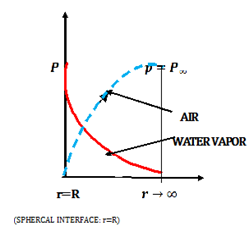Figure 1. Variation of partial pressures of vapor and air

2.1 Thermal process in the seed

For a spherical shape of the seed the unsteady state thermal conduction is defined by the following equation

$\frac{\partial}{\partial r}\left[r^{2} \frac{\partial T}{\partial r}\right]=\frac{1}{\alpha}\left[\frac{\partial}{\partial \tau}\left(r^{2} T\right)\right]$       (1)

The seed is subjected to boundary conditions as follows:

$r \rightarrow \infty ; T=T_{g}=$cons $\tan t : P=P_{\infty}=$constant

$r=R : T=T_{i}(\tau)$

$\tau=0 :(0<r<R) ; T=T_{0}$

For $\tau>0 : A t r=R$          (2)

The convective heat transfer at the periphery of the spherical interface of the seed is given by the Eq(3). as follows:

$-k\left[\frac{\partial T}{\partial r}\right]_{r=R}=h_{c}\left(T_{g}-T_{i}(\tau)\right)$         (3)

It is required to find $T=T_{i}(\tau)$ for convective thermal boundary condition (3) from the unsteady conduction Eq (1).

2.2 Vapor mass diffusion equation

Mass transfer of water vapor from the spherical interface i.e. at r=R can be defined as follows according to the model shown in Figure 1.

$-\frac{d M}{d \tau}=4 \pi R^{2} h_{m}\left(\rho_{v(r=R)}-\rho_{i \infty}\right)$        (4)

However, as per the model (from Figure 1)

$P_{i v}=P_{\infty}$ at $r=R$

$P_{i v}=0$ at $r \rightarrow \infty \Rightarrow \rho_{i \infty}=0$

Thus,

$\left[\rho_{v(r=R)}\right]=\left[\frac{P_{\infty}}{R_{0}\left[T_{i}(\tau)+273\right]}\right]$ and $\left[\rho_{v({\infty})}=0\right]$

Hence, Eq(4) transforms into

$-\frac{d M}{d \tau}=4 \pi R^{2} h_{m}\left(\rho_{v(r=R)}\right)=4 \pi R^{2} h_{m}\left[\frac{P_{\infty}}{R_{0}\left[T_{i}(\tau)+273\right]}\right]$       (5)

The following dimensionless parameters are introduced into the Eqs (1), (2), (3) and (4).

$R^{+}=\left[\frac{r}{R}\right] ; \quad T^{+}=\frac{T}{T_{g}}$

$F o=\left[\frac{\alpha \tau}{R^{2}}\right]$

Eq(1) transforms to

$\frac{\partial}{\partial R^{+}}\left[R^{+2} \frac{\partial T^{+}}{\partial R^{+}}\right]=\left[\frac{\partial}{\partial F_{O}}\left(R^{+2} T^{+}\right)\right]$         (6)

With boundary conditions

$0<R^{+}<1 : T^{+}=T^{+}(F o)$

$F o>0 : R^{+}=1 ; T^{+}=T_{i}^{+}(F o)$

$R^{+}=0 : F o>0 : T^{+}=T_{c}^{+}(F o)$

$F o=0 :\left(0<R^{+}<1\right) : T^{+}=\frac{T_{o}}{T_{g}}=T_{o}^{+}$

$F o>0 :$ at $R^{+}=0 ; T^{+}=T_{c}^{+}$

Further at $R^{+}=1 : F o>1$

$\left[\frac{\partial T^{+}}{\partial R^{+}}\right]_{R^{+}=1}=B i\left(1-T_{i}^{+}(F o)\right)$     (7)

Eq(5) in dimensionless form can be written as follows

$\frac{\partial M^{+}}{\partial F_{O}}=-\pi_{m}\left[\frac{\left(T_{g}+273\right)}{273+T_{g} T_{i}^{+}}\right]$       (8)

where $M^{+}=\frac{M(\tau)}{M_{o}}$

3. Solution Procedure

The unsteady state conduction heat Eq (6) & mass diffusion Eq (8) are solved simultaneously to find drying rate of the seeds with respect to time with the boundary condition for solving Eq (8) as

$F o=0 : M^{+}=1$     (9)

The solution is excepted to be in the form of

$M^{+}=f\left[B i, \pi_{m}, F o, \frac{T_{i}}{T_{g}}\right]$       (10)

Thus, the objective of the study is to establish the correlation i.e. Eq (10)

The solution is obtained by Integrating Eq (6) between the limits R+=0: R+=1 it for the condition R+≠R+(Fo)

$\frac{d}{d F_{O}} \int_{R^{*}=0}^{R^{*}=1} T^{+} R^{+2} d R^{+}=\left[\frac{\partial T^{+}}{\partial R^{+}}\right]_{R^{+}=1}$         (11)

For Fo>0, the following thermal profile for T+ can be assumed satisfying the boundary conditions both at R+=0 and R+=1. Further it satisfies smoothening conditions at R+=0.

$T^{+}=\left(T_{i}^{+}-T_{c}^{+}\right) R^{+4}-T_{c}^{+}$        (12)

Substitution of Eq(12) into Eq(11) yields

$\frac{1}{7} \frac{d T_{i}^{+}}{d F_{O}}+\frac{4}{21} \frac{d T_{c}^{+}}{d F_{O}}=4\left[T_{i}^{+}-T_{c}^{+}\right]$      (13)

The boundary conditions are Fo=0:

$T_{i}^{+}=T_{c}^{+}=T^{+}(0)$ prescribed input

Substitution of Eq(12) into Eq(7) after simplification yields

$T_{i}^{+}=\frac{T_{c}^{+}+0.25 B i}{1+0.25 B i}$      (14)

Solving Eqs (13) and (14) together the following variations of thermal conditions at the extreme salient points of center and spherical surface can be established for Fo>0

$T_{i}^{+}=1-\left[1-T_{i}^{+}(0)\right] \operatorname{Exp}\left\{-84 \frac{B i}{7+B i} F o\right\}$      (15)

$T_{c}^{+}=T_{i}^{+}[1+0.25 B i]-0.25 B i$      (16)

3.1 Solution of Eq(8)

It can be accomplished by expressing Eq(8) in finite difference form with the established solution i.e., Eq (15)

$M^{*}(I+1)=M^{*}(I)-\Delta F o^{*} \pi_{m}$     (17)

$\Delta F o=F o R / J$

$M^{*}(I=1)=1$

Thus, the Variation of M (I), with 0 < I < J can be established to obtain a correlation Eq. (10).

4. Results and Discussion

Thus non–dimensional Eqs(15), (16) and (17) are programmed to obtain typical results using FORTRAN software and are presented in graphical form in the Figure 2 to Figure 9.

## 2.png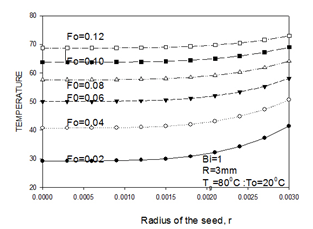Figure 2. Temperature variation within the seed with Time

## 3.png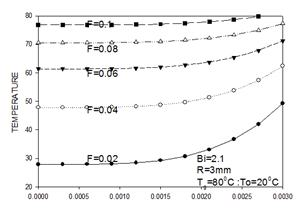## 3a.png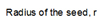Figure 3 Temperature variation within the seed with Time

## 4.png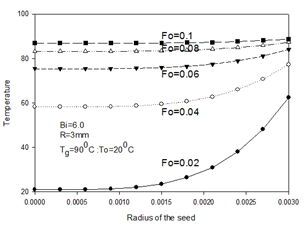Figure 4 Temperature variation within the seed with Time

Figures (2, 3 and 4) show the temperature distribution as a function of radius and time inside the seed. Since the heat transfer took place from the outer surface to the center of the seed the temperature of the seed at its center is kept increasing gradually with increasing for number before it attains its equilibrium value after some time. It is also observed that with increasing Biot number the temperature gradient within the seed also increases as it offers more resistance to conduction heat transfer. It is also clear that the temperature gradually decreases from the interface of the seed to its center.

## 5.png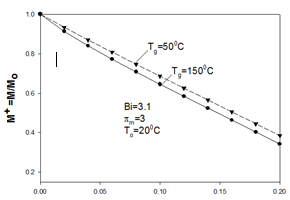## 5a.png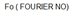Figure 5. Variation of M+ with ambient temperature

Figure 5 shows the rate of evaporation of moisture with respect to time until it reaches its equilibrium value for various hot medium conditions. It is observed that with increasing hot medium temperature the rate of evaporation also increases.

## 6.png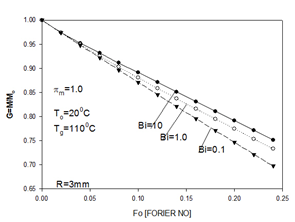Figure 6. Effect of Biot modulus on dehydration

Figure 6 shows the effect of Biot modulus on rate of evaporation of moisture from the seed. It is observed that at lower Biot number the rate of evaporation high due to relatively low internal resistance offered by the seed.

Figure 7 shows the temperature variation of the seed at its center and at its surface. At higher Biot number the difference in temperature at center and surface is found high at any particular time interval.

## 7.pngFigure 7. Variation of temperature of seed at center and at interface

## 8.png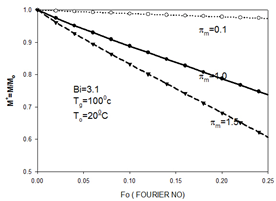Figure 8. Effect of mass transfer parameter on dehydration rate

Figure 8 shows the effect of Mass transfer parameter on rate of dehydration. It is obvious from the figure with increase in convective mass transfer coefficient i.e mass transfer parameter the rate of dehydration is also found increased.

4.1 Correlation for rate of evaporation

With the aid of the program results are obtained for the following range of parameters.

$70^{\circ} \mathrm{C}<T_{g}<105^{\circ} \mathrm{C} ; 15<T_{o}<25$

$0.4<\pi_{m}<1.75 ; 0.89<B i<50 ; 0.2<F o<1.2$

$\frac{M}{M_{o}}=1-0.91\left[\frac{273+T_{o}}{273+T_{g}}\right]^{-0.525} \pi_{m}^{0.99} B i^{-0.048} F_{O}^{0.968}$     (18)

The correlation to determine the rate of evaporation, Eq(18) is obtained for 155 data obtained from the program by applying regression with an average deviation of 1.073% and a standard deviation of 1.406%.

Further, Eq (18) is validated in Fig. 9

## 9.png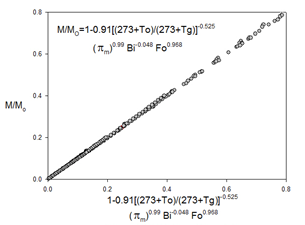Figure 9 Validation of correlation

5. Conclusions

The following conclusions can be drawn from the study

1. The rate of increase of temperature within the seed during first few minutes is rapid.
2. The variation in temperature at the surface of the seed and at the center of the seed is negligible at lower Biot numbers.
3. The rate of moisture loss from the seed is found linear w.r.t time.
4. With increase in mass transfer parameter the rate of drying of the seed also increased.
5. With increasing in internal conduction resistance of the seed the rate of drying of the seed decreased.
6. The rate of evaporation of moisture exposed to hot dry medium can be calculated using the following equation

$\frac{M}{M_{o}}=1-0.91\left[\frac{273+T_{o}}{273+T_{g}}\right]^{-0.525} \pi_{m}^{0.99} B i^{-0.048} F o^{0.968}$

Nomenclature
 A Area, m2 Bi Biot Number, hcR/k, dimensionless C Moisture concentration,mol.m-3 Fo hc Fourier Number, at/r2, dimensionless Convective heat transfer coefficientWm-2K hm Convective mass transfer coefficient ms-1 k Thermal conductivity Wm-1K-1 M Moisture Content, kg P Partial Pressure, Nm-2 R Radial dimension at time t Ro Universal gas constant, Jkg-1mol-1K-1 T Temperature, K Greek symbols $\alpha$ Thermal Diffusivity, m2s-1 $\delta$ Differential operator $\rho$ Density, kgm-3 $\pi_{\mathrm{m}}$ Mass Transfer Parameter $\left(\frac{4 \pi R^{4} h_{m} P_{\infty}}{R_{o} \alpha M_{o} T_{o}}\right)$ $\tau$ Time, s Subscripts $\infty$ Free stream I Interface m Mass o Intial at $\tau$ =0 Superscripts + Non-dimensional v Vapor
Appendix

In nature, we find mostly two geometries in the seeds:

1. Spherical shape
2. Ellipsoidal shape

Our analysis holds well both for spherical and non-spherical seeds when the equivalent diameter is considered for ellipsoidal seeds. The analysis is formulated for spherical geometries. However, for ellipsoidal geometry the equivalent diameter as a first approximation is to be employed.

1.Spherical seed

## 10.png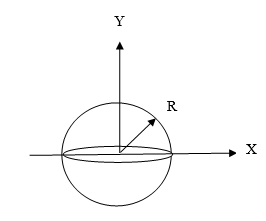VOLUME $=\frac{4}{3} \pi \mathrm{R}^{3}$ :   D=2R

2. Ellipsoidal seed - (equivalent diameter)

## 11.png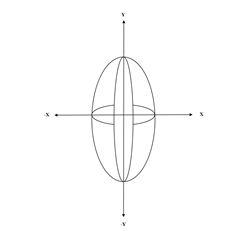where the geometry of the seed is defined by the coordinate system $(-a<X<a) :(-b<Y<b)$

Volume of the seed = $\int_{-b}^{b} \pi x^{2} d y$

where $x^{2}=a^{2}\left(1-\frac{y^{2}}{b^{2}}\right)$

Volume = $\frac{4}{3} \pi b^{2} a$

On simplification $D_{e q}=2\left(b^{2} a\right)^{1 / 3} \mathrm{Y}$

Define for non- spherical seed

$D=D_{e q}=2\left[b^{2} a\right]^{1 / 3}$

For spherical geometry b=a=R: Deq= 2R

References

 Luikov A.V. (1966). Heat and Mass Transfer in capillary-Porous Bodies, Pergamon Press, New York. DOI: 10.1002/aic.690130305

 Muthukumarappan K., Gunasekaran S. (1996). Finite element simulation of corn moisture adsorption, Transactions of the ASAE, Vol. 39, No. 6, pp. 2217–2222. DOI: 10.13031/2013.27728

 Ranjan R., Irudayaraj J., Juna S. (2001). Three-dimensional control volume approach to modeling heat and mass transfer in food materials, Transactions of the ASAE, Vol. 44, No. 6, pp. 1975–1982. DOI: 10.13031/2013.6996

 Agarwal K.K., Clary B.L., Schroeder E.W. (1973). Mathematical models of peanut pod geometry, Transactions of the ASAE, Vol. 16, No. 2, pp. 315–319. DOI: 10.13031/2013.37510

 Haghighi K., Irudayaraj J., Stroshine R.L., Sokhansanj S. (1990). Grain kernel drying simulation using the finite element method, Transactions of the ASAE, Vol. 33, No. 6, pp. 1957–1965. DOI: 10.13031/2013.31564

 Manal H., Al-Hafidh S.M.A. (2008). Heat and mass transfer during air drying of fruits, The Iraqi Journal for Mechanical and Material Engineering, Vol. 8, No. 2, pp. 110–126.

 Aprajeeta J., Gopirajah R., Anandharamakrishnan C. (2015). Shrinkage and porosity effects on heat and mass transfer during potato drying, Journal of Food Engineering, Vol. 144, pp. 119–128. DOI: 10.1016/j.jfoodeng.2014.08.004

 Stanescu G., Risso M. (2016). Optimization of continuous mixed-flow grain dryers by constructal theory, International Journal of Heat and Technology, Vol. 34, Special Issue 1, pp. S151-S160. DOI: 10.18280/ijht.34Sp0120

 Mihoubi D., Bellagi A. (2008). Two-dimensional heat and mass transfer during drying of deformable media, Applied Mathematical Modeling, Vol. 32, No. 3, pp. 303–314. DOI: 10.1016/j.apm.2006.12.003

 Aviara N.A., Ajibola O.O., Oni S.O. (2004). Sorption equilibrium and thermodynamic characteristics of soya bean, Biosystems Engineering, Vol. 87, No. 2, pp. 179–190.

 Muchilwa I., Hensel O., Matofari J.W. (2014). Evaluating the water activity simulation consistency of empirical models for shelled and cobed maize drying, International Journal of Agricultural Sciences, Vol. 4, No. 3, pp. 177–188.

 Vega A., Fito P., Andre A., Lemus R. (2007). Mathematical modeling of hot-air  drying  kinetics  of

red bell pepper, Journal of Food Engineering, Vol. 79, No. 4, pp. 1460–1466. DOI: 10.1016/j.jfoodeng.2006.04.028

 Lorenzini G., Saro O. (2016). Analysis of water droplet evaporation through a theoretical-numerical model, International Journal of Heat and Technology, Vol. 34, Special Issue 2, pp. S189-S198. DOI: 10.18280/ijht.34Sp0201

 Oleg A.K. (2012). Experimental study and mathematical model for soya bean drying in thin layer, Systems Engineering, Vol. 113, No. 6, pp. 54–64.

 Perea-Floresa. M.J., Garibay-Feblesb V., Chanona-Pereza J.J., Calderon-Domingueza G., Mendez-Mendezc J.V., Palacios-Gonzalezb E., Gutierrez-Lopeza. (2012). Mathematical modelling of castor oil seeds drying kinetics in fluidized bed at high temperatures, Industrial Corps and Products, Vol. 38, pp. 64–21. DOI: 10.1016/j.indcrop.2012.01.008

 Kamyar H., Segerlind L.J. (1991). Modeling simultaneous heat and mass transfer in an isotropic sphere-a finite element approach, Transactions of the ASAE, Vol. 31, No. 2, pp. 629–637. DOI: 10.13031/2013.30758